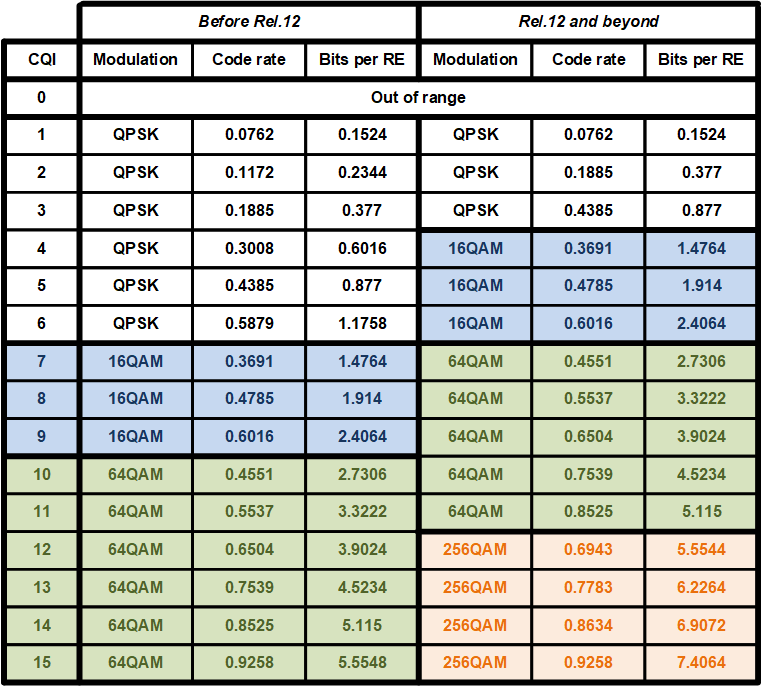﻿ LTE Modulation and Coding Scheme (MCS)

# LTE Modulation and Coding Scheme (MCS)

MCS (Modulation and Coding Scheme) defines how many useful bits can be transmitted per Resource Element (RE). MCS depends on radio link quality. The better quality the higher MCS and the more useful data can be transmitted. And vice versa the worse radio conditions the lower MCS and the less useful data can be transmitted. In other words MCS depends on error probability. Typically there is error probability threshold defined that equal to 10%. To maintain error probability not more than this value in varying radio condition MCS is adjusted accordingly. In LTE it's done once per TTI (1 ms) individually for each active user.

MCS defines the following two aspects:
• Code rate
• Modulation

### Code rate

In LTE Turbo encoder is used with 1/3 code rate (i.e. there are three times more bits after Turbo encoder than before). However real ratio of useful bits to total transmitted bits (useful bits plus parity bits) depends on actual radio link quality as not all data generated by Turbo encoder can be transmitted (just as much as needed to perform data transmission with error probability less than 10%). In fact coding rate range is 0.0762 - 0.9258. Radio link quality is estimated based on CQI (Channel Quality Indicator). CQI is reported by UE (User Equipment) and measured based on Cell Reference Signal (CRS). The better radio conditions the higher CQI and the higher coding rate (=the higher data rate). See a table below for further details.

### Modulation

Modulation defines how many bits can be carried by a single RE (irrespective whether it's useful or parity bits). In LTE there are following modulations supported: QPSK, 16QAM, 64QAM and 256QAM. With QPSK there are 2 bits can be transmitted per RE, with 16QAM - 4 bits, with 64QAM - 6 bits and with 256QAM - 8 bits. Finally we have the following table to map CQI value to modulation and coding rate.In case of MIMO usage 'Bits per RE' column should be multiplied by number of data streams to get a final value.

Also see how to calculate LTE throughput using MCS and LTE throughput calculator.

You can share your feedback, questions and/or proposals sending an email to alexey.anisimov86[at]gmail.com

© Alexey Anisimov, 2019